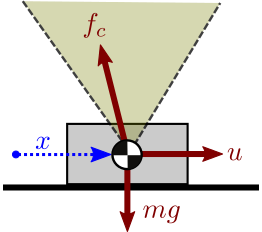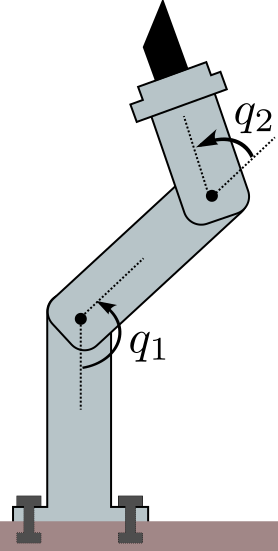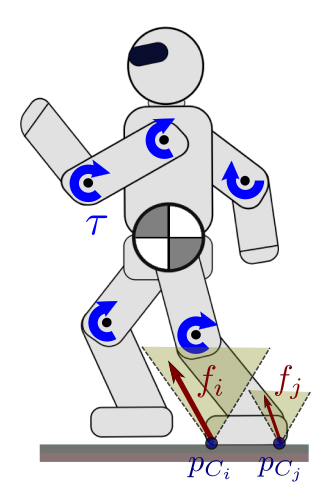# Equations of motion

It is common to see the equations of motion of manipulators or humanoids reminded in the preamble of research papers. Their origin can be tracked down in textbooks, where they are derived from Newtonian or Lagrangian mechanics. In this tutorial, we will see what are the equations of motion for manipulators and mobile robots, and how they can be computed in practice.

## Generalities

The equations of motion describe the motion of a physical system as a function of time and controls. In their most general form, they are written:

\begin{equation*} F(\q(t), \qd(t), \qdd(t), \bfu(t), t) = 0, \end{equation*}

where

• $$t$$ is the time variable,
• $$\q$$ is the vector of generalized coordinates, for instance the vector of joint-angles for a manipulator,
• $$\qd$$ is the first time-derivative (velocity) of $$\q,$$
• $$\qdd$$ is the second time-derivative (acceleration) of $$\q,$$
• $$\bfu$$ is the vector of control inputs.

These equations provide a mapping between the control space (the commands that we send to actuators) and the state space of the robot (what we want to control in practice: the robot's position and velocity).

### Illustration

As a simple example, imagine a block sliding on a table, which we can see as a 1-DOF system with coordinate $$x$$. The operator applies a horizontal force $$u$$ that pushes the block forward.If the force is high enough to overcome friction, applying Newton's second law of motion (and Coulomb's model of sliding friction) we get: $$m \xdd = u - \mu_k m g,$$ where $$\mu_k$$ is the kinetic coefficient of friction. This expression is of the form $$F(x, \xd, \xdd, u, t) = 0.$$

### Assumptions

In robotics, we make the following assumptions:

• Rigid bodies: the robot consists of a set of rigid bodies, called "links", connected by actuated joints. This assumption allows us to use the theory of rigid body dynamics, which is central to both manipulator and humanoid robotics.
• D'Alembert's principle: internal forces  are conservative. This assumption is equivalent to Gauss's principle of least action.

## Case of manipulatorsManipulators are a particular type of articulated system where at least one link is fixed to the environment. We suppose that the fixation is strong enough to withhold any effort exerted on it. Under these assumptions, the equations of motion for a manipulator can be written:

\begin{equation*} \bfM(\bfq) \qdd + \qd^\top \bfC(\bfq) \qd + \bfg(\bfq) = \bftau \end{equation*}

where, denoting by $$n = \dim(\bfq)$$ the degree of freedom of the robot,

• $$\bfM(\q)$$ the $$n \times n$$ inertia matrix,
• $$\bfC(\q)$$ the $$n \times n \times n$$ Coriolis tensor,
• $$\bfg(\q)$$ the $$n$$-dimensional gravity vector,
• $$\bftau$$ the $$n$$-dimensional vector of actuated torques.

This expression is simpler than the general form $$F(\q, \qd, \qdd, \bfu, t) = 0$$ in a number of ways. One of them is that it is time-invariant: given that the robot is in a state $$(\q, \qd)$$ and we apply a torque $$\bftau,$$ the resulting joint accelerations $$\qdd$$ will be the same regardless of $$t.$$ 

### Computation of the inertia matrix

Another significant point is that the matrices and tensors of the formula above can be computed by inverse dynamics. For instance, in OpenRAVE one can use Robot.ComputeInverseDynamics to compute each of the three terms

\begin{equation*} \begin{array}{ccc} m := \bfM(\bfq) \qdd & c := \qd^\top \bfC(\bfq) \qd & g := \bfg(\bfq). \end{array} \end{equation*}

By setting $$\qdd$$ to the vector $$\bfe_i$$ of the canonical basis of $$\mathbb{R}^n$$, one can then compute the $$i^{\textrm{th}}$$ line of the inertia matrix $$\bfM(\q).$$ This is known as the unit vector method, which was proposed by Walker and Orin in [Walker1982]. Here is a Python sample:

def compute_inertia_matrix(robot, q, external_torque=None):
n = len(q)
M = zeros((n, n))
with robot:
robot.SetDOFValues(q)
for (i, e_i) in enumerate(eye(n)):
m, c, g = robot.ComputeInverseDynamics(
e_i, external_torque, returncomponents=True)
M[:, i] = m
return M


Note how there is no need to set the robot's velocity in the function above, as ComputeInverseDynamics computes separately the three terms m, c and g.

## Case of mobile robotsContrary to manipulators, mobile robots such as humanoids can make or break contacts with the environment. To fully describe their position in space, it becomes necessary to add six coordinates to the vector of joint angles, accounting for the position and orientation of a base link with respect to an inertial frame. If the robot has $$n$$ degrees of freedom, this means that $$\q \in \mathbb{R}^{n + 6}$$ . As the vector of actuated torques is still $$\bftau \in \mathbb{R}^n$$, humanoids are underactuated systems: they cannot control directly all their degrees of freedom.

The contact constraint between a manipulator and the environment was implicitely enforced, as the vector of joint angles was independent from the world position of the robot. With mobile robots it is not the case any more, and contacts need to be actively enforced by contact constraints.

### Point contacts & contact forces

Kinematically, a point contact constraint is an equality of the form

\begin{equation*} \bfp_{C}(\q) = \bfp_{D}, \end{equation*}

where $$C$$ is the position of a robot's point (in the inertial frame) and $$D$$ is a fixed point (in the inertial frame). It binds positions, but also velocities and accelerations:

\begin{equation*} \begin{array}{rcl} \bfpd_C = 0 & \Leftrightarrow & \bfJ_C \qd = \boldsymbol{0} \\ \bfpdd_C = 0 & \Leftrightarrow & \bfJ_C \qdd + \qd^\top \bfH_C \qd = \boldsymbol{0} \end{array} \end{equation*}

where $$\bfH_C$$ is hessian of the position vector $$\bfp_C(\q).$$ Assuming the humanoid is making $$N$$ point contacts $$C_1, \ldots, C_N$$ with the environment, their equations of motion become:

\begin{equation*} \bfM(\bfq) \qdd + \qd^\top \bfC(\bfq) \qd + \bfg(\bfq) = \bfS^\top \bftau + \sum_{i=1}^N \bfJ_{C_i}^\top \bff_i, \end{equation*}

where:

• $$\bfS$$ is the $$n \times (n + 6)$$ matrix that selects the $$n$$ actuated coordinates in $$\q$$. If the six base-link coordinates are the last entries of $$\q,$$ then $$\bfS = \left[ \bfE_n \ {\boldsymbol{0}}_{6 \times n} \right]$$.
• $$\bfJ_{C_i}$$ is jacobian of the position vector (in the inertial frame) $$\bfp_{C_i}(\q)$$ of a contact point $$C_i.$$
• $$\bff_i$$ is the contact force exerted by the environment on the robot at the contact point $$C_i.$$

About the origin of the new term $$\bfJ_C^\top \bff$$, see e.g. [Spong2004] (p. 272).

Contact points $$C_i$$ are taken at the interface between robot's links and the environment. In the case of surface contacts, when a robot's surface is in full contact with an environment surface (such as a foot on the floor), it is sufficient to take $$C_i$$'s at the vertices of the contact polygon [Caron2015].

### Surface contacts & contact wrenches

Surface contacts (such as a flat foot on the floor) can be modeled by using three or more point contacts, yet at the cost of a redundant representation, which can e.g. prevent the application of time-optimal retiming or other methods that required non-redundant force variables. A non-redundant alternative to this is to use frame contact constraints.

Kinematically, a frame contact constraint is an equality

\begin{equation*} {}^{D}\bfT_{C}(\bfq) = \bfE_4, \end{equation*}

where $${}^{D}\bfT_{C}$$ is the transform matrix between a robot's frame with origin at $$C$$ and an inertial frame with origin at $$D$$ . It binds velocities and accelerations as well by:

\begin{equation*} \begin{array}{rcl} \frac{\rm d}{\d{t}} {}^D\bfT_C = \boldsymbol{0}_{4 \times 4} & \Leftrightarrow & \calJ_C \qd = \boldsymbol{0} \\ \frac{\rm d^2}{\d{t}^2} {}^D\bfT_C = \boldsymbol{0}_{4 \times 4} & \Leftrightarrow & \calJ_C \qdd + \qd^\top \calH_C \qd = \boldsymbol{0} \end{array} \end{equation*}

where $$\calJ_C$$ is the manipulator jacobian of the contact frame rooted at $$C$$, obtained by stacking:

• the rotational jacobian of the contacting link, i.e., the matrix $$\bfJ^{\textrm{rot}}$$ such that the rotational velocity $$\omega$$ of the link satisfies $$\omega = \bfJ^{\textrm{rot}} \qd$$ (in OpenRAVE, Robot.ComputeJacobianAxisAngle); and
• the linear jacobian $$\bfJ_C$$, i.e., the matrix such that the linear velocity $$\bfpd_C$$ satisfies $$\bfpd_C = \bfJ_C \qd$$ (in OpenRAVE, Robot.ComputeJacobianTranslation).

Under frame contact constraints, the equations of motion become:

\begin{equation*} \bfM(\bfq) \qdd + \qd^\top \bfC(\bfq) \qd + \bfg(\bfq) = \bfS^\top \bftau + \sum_{i=1}^N \calJ_{C_i}^{\top} \bfw_{C_i}, \end{equation*}

where $$\bfw_{C_i} = [\bftau_{C_i}\ \bff_i]$$ is the contact wrench exerted by the environment on the robot at the link $$i$$ to which $$C_i$$ belongs. See Chapter 2 of [Featherstone2008] for an excellent introduction to wrenches and, more generally, to spatial vector algebra. The order of $$\bftau_{C_i}$$ and $$\bff_i$$ does not matter when taking the vector representation of a wrench. However, here it needs to be consistent with the structure of the manipulator jacobian: if the rotational jacobian is stacked first, then the resultant moment needs to come first in the wrench.

## Footnotes

  Internal forces, also known as constraint forces, are the forces acting at each joint of the robot to prevent undesired motions. Consider two bodies A and B connected by a rotary joint, actuated with torque $$\tau.$$ Unconstrained, the relative position of A with respect to B has six DOF. Constrained by the joint, it has one remaining DOF, and five DOC. The torque $$\tau$$ actuates the degree of freedom, while a five-dimensional internal force is created by the degrees of constraint.
  Formally, time-invariance means that the equations of motion can be written $$F(\q, \qd, \qdd, \bfu) = 0$$, i.e., with no explicit dependency on the time-variable $$t$$.
  In OpenRAVE, these six degrees of freedom can be added via six joints between "mimic" bodies connecting the robot to a world origin; see for instance how the Robot class is implemented in pymanoid.
  For definitions, a clear and concise introduction to robot kinematics can be found in Chapter 2 of [Kajita].
Pages of this website are under the CC-BY 4.0 license.